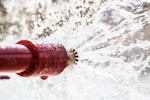## Why does Pipe Flow Expert use the Darcy-Weisbach equation rather than the Hazen-Williams equation?

The Pipe Flow Expert software uses the Darcy-Weisbach equation to calculate flow rates and pressure drops in pipes. Friction factors are calculated using the Colebrook-White formula. These formulae give very accurate results for Newtonian fluids (i.e. non-compressible liquids such as water) and they are much more accurate than the simpler Hazen-Williams method.

There are various NFPA standards and documents. Some are older and some are newer. Some refer to different types of systems.One of the old NFPA standards states that the Hazen-Williams method should be used to perform the friction loss calculations for water-based sprinkler systems. We intentionally did not include it within the Pipe Flow Expert software because the Darcy-Weisbach equation with Colebrook-White friction factors is much more accurate (although more complicated to calculate).

The NFPA have not yet updated all of their standards to reflect current best practice which would specify a requirement to use the Darcy-Weisbach method as the new standard for all calculations. The Darcy-Weisbach calculation is now specified as a requirement by the NFPA for some cases: it just depends where you are and who is reading which rules and standards, and who is going to be approving your design. We believe that the Darcy-Weisbach calculation will be accepted for all designs.

For example, see page 3 in this document from the NFPA, which states , ‘23.4.2.1.3 For antifreeze solutions systems greater than 40 gal (151 L) in size, the friction loss shall also be calculated using the Darcy–Weisbach formula’

Hazen Williams is really only used with Water and for other fluids (foams, anti-freeze fluid, etc.) the Darcy-Weisbach equation should be used. Even with water, Hazen Williams does not give accurate results when compared to the results from the Darcy-Weisbach equation.

Further information about the different methods of calculating friction loss is given in a Report from the Viking Group that explains why the Darcy-Weisbach equations should be used (is required) in certain situations.

We would recommend using the Darcy-Weisbach equation to calculate friction loss in all cases for a system with a none compressible Newtonian fluid (which includes water).

Secure Online PaymentsPipe Flow Software: Piping design, Pressure drop calculator, Flow rate calculator, Pump head calculations, Pump selection software.  Copyright © Pipe Flow Software 1997-2020